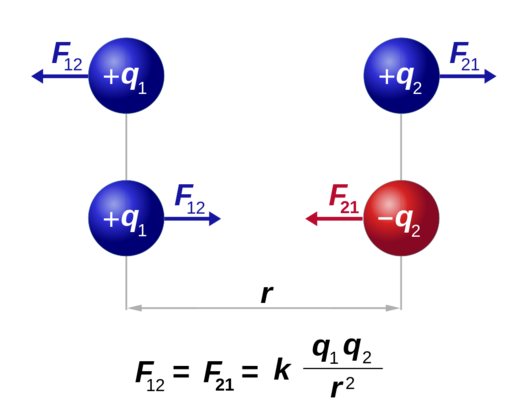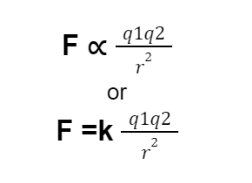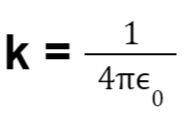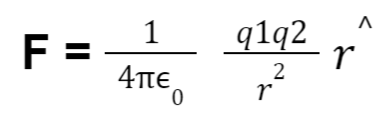HS-PS2-4

# Coloumb’s Law Study Guide

Coulomb's law describes the action of the electric force on charged particles. This article explains how it works.

# INTRODUCTION

Everybody knows the balloon party trick- rub it over someone’s dry hair, and it sticks to the wall, the dog’s hair, and even bends a small stream of water! You may already know that rubbing a balloon over certain materials “charges” it up, but what really is happening at the atomic level? What is this force that attracts or repels charged objects? What are the physics principles that determine their behavior? Let’s find out!Source

## ELECTRIC FORCE AND COULOMB’S LAW

Even the most basic experiments, such as the one with charged balloons, show that two objects with an electric charge exert a force on each other. Objects with the same charge repel while objects with the opposite charge attract. That brings up an interesting question- what determines the force exerted by the charge, and how is it affected by the medium between the objects?

The answer to that comes from Coulomb's law.Source

If q₁ and q₂ are two charges separated by a distance r, then according to Coulomb's law the electric force magnitude is directly proportional to the net charge on the object and inversely proportional to the distance between the charges.

Mathematically:F is the electric force between the two charges

k is the constant of proportionality called the Coulomb’s constant.

The value of k= 8.99×10⁹ Nm²/C².

We can see from the equation above that Coulomb's law is an example of the inverse-square law, according to which the magnitude of the force is proportional to the square of the denominator. In other words, the force decreases greatly as the distance between the charges increases.

## HOW MUCH FORCE DOES 1 COULOMB OF CHARGE EXERT

The SI unit of charge is expressed as coulomb (C). A coulomb is the charge of 6.25 x10¹⁸ electrons.

The charge on a single electron is 1.6 x 10⁻¹⁹ C. This is known as the elementary charge. A proton holds the same charge but has the opposite sign. Now here’s the incredible part, if you can get one coulomb of charge each to about 1 meter apart in a vacuum, the charges would apply a force of 9x10⁹ N!! If they are of the same polarity, they would repel at that force and if they are of the opposite polarity, they would attract.

## PERMITTIVITY

When dealing with the electrical force applied by charges, it is important to understand that the medium in which the charges are present influences the force. To quantify this influence a term called permittivity (0) is used.

The coulomb’s constant k is also represented as:Hence coulomb’s law in vector form can be written as:where,

F = electrical force

0 = vacuum permittivity at 8.86 × 10⁻¹² F.m⁻¹

q₁, q₂ = charges

r = separation between charges

r^ = unit vector in the radial direction of the charges

# CONCLUSION:

• Electric charges exert an attractive or repulsive force on each other.
• Opposite charges repel while like charges attract.
• According to coulomb’s law the electric force magnitude is directly proportional to the net charge on the object and inversely proportional to the distance between the charges.

## FAQs:

1. What is Coulomb's law?

According to Coulomb's law the electric force magnitude is directly proportional to the net charge on the object and inversely proportional to the distance between the charges.

2. What is meant by 1 coulomb?

One coulomb is the charge of 6.241 x 10¹⁸ electrons.

We hope you enjoyed studying this lesson and learned something cool about Coulomb’s law! Join our Discord community to get any questions you may have answered and to engage with other students just like you! We promise, it makes studying much more fun! 😎

## SOURCES:

1. Coulomb’s Law. https://flexbooks.ck12.org/cbook/ck-12-physics-flexbook-2.0/section/15.3/primary/lesson/coulomb%E2%80%99s-law-phys/. Accessed 22 Feb 2022.
2. Coulomb’s Law. https://www.physicsclassroom.com/class/estatics/Lesson-3/Coulomb-s-Law. Accessed 22 Feb 2022.
3. Coulomb’s Law. https://byjus.com/jee/coulombs-law/. Accessed 22 Feb 2022.
4. Coulomb's law. https://openstax.org/books/physics/pages/18-2-coulombs-law. Accessed 22 Feb 2022.